## 5t-9=-3t+7. I need help this is equations variables both sides

Question

5t-9=-3t+7. I need help this is equations variables both sides

in progress 0
7 months 2021-07-21T20:20:24+00:00 2 Answers 3 views 0

1. Hey there!☺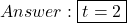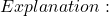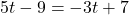Let’s start by adding 3t to both sides of the equation.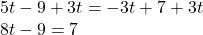Now let’s add 9 to both sides of the equation.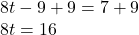Now let’s divide both sides by 8.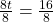Now let’s simplify 16/8.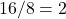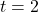Hope this helps!

t = 8

Step-by-step explanation:

5t – 3t – 9 = 7

5t – 3t = 7 + 9

2t = 7+ 9

2t = 16    <- divide